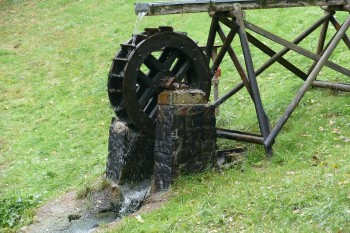# Nuclear Energy# Mechanical energyMechanical energy can be defined as the ability to produce a mechanical work that a body possesses due to causes of mechanical origin, such as its position or its speed. There are two forms of mechanical energy that are kinetic energy and potential energy.

The mechanical energy of a body is the sum of its kinetic energy and its potential energy. Mechanical energy can be partially transformed into other types of energy, such as electrical energy (in a ventilator), and can be obtained by transforming other energies, such as chemical energy (in a person who walks).

The kinetic energy of a body is associated with its speeds, linear and angular, and its mass). Actually, kinetic energy is the energy you are using at a given moment. Potential energy, as the word itself indicates, is the energy that you can potentially obtain. For example, if we hold a ball with the hand it has the potential energy equivalent to the kinetic energy that it would have if we let it go. If we release the ball and fall, it will start to acquire speed and therefore kinetic energy. As the kinetic energy increases, the potential energy decreases. In this case, the mechanical energy always remains constant.

## Conversion of mechanical energy

Nowadays, many technological devices and machines convert mechanical energy into other forms of energy or vice versa. Of these devices we highlight the following categories:

• An electric motor converts electrical energy into mechanical energy. By supplying electric current to the motor we obtain a mechanical movement, the rotation of an axis. This axis, being an element with a certain mass and speed (angular velocity), has kinetic energy and, therefore, mechanical energy.
• A generator converts mechanical energy into electrical energy. It is the same case of the electric motor, but in the opposite direction.
•A hydroelectric power plant converts the mechanical energy of water into a storage dam into electrical energy. Before opening the gates, all the water accumulated behind the gate has potential energy. When opening the gate, the water comes out under pressure with a certain speed, therefore, it becomes kinetic energy. The outgoing water interacts with a turbine that will be responsible for converting the kinetic energy into electrical energy. It's what we call hydraulic power.
• An internal combustion engine is a thermal engine that obtains mechanical energy from chemical energy by burning fuel (diesel, gasoline, etc.). In the case of a car, this mechanical energy is used to travel. In the case of a thermal power plant, it is used.
• A steam engine converts the thermal energy of steam into mechanical energy. In this case you can also analyze how the steam is obtained. To convert water into steam you need heat energy, this energy can be obtained in many ways, by solar thermal energy (unusual in these cases) or with the combustion of fossil fuels such as coal, for example.
• A turbine converts the kinetic energy of a gas or liquid stream into mechanical energy. As we have said in the case of hydraulic energy.

## Distinction of other types of energy with mechanical energy

The classification of energy in different types often follows the limits of the fields of study in the natural sciences.

Chemical energy is the type of potential energy stored in chemical bonds and is studied in chemistry. An example is gunpowder. Providing a bit of heat to the gunpowder produces an exothermic chemical reaction, that is, with energy release to the outside.

Nuclear energy is energy stored in the interactions between particles in the atomic nucleus and is studied in nuclear physics.

Electromagnetic energy is in the form of electric charges, magnetic fields and photons. It is studied in electromagnetism.

Various forms of energy in quantum mechanics; for example, the energy levels of electrons in an atom.

valoración: 3 - votos 1

Last review: August 22, 2018

Back